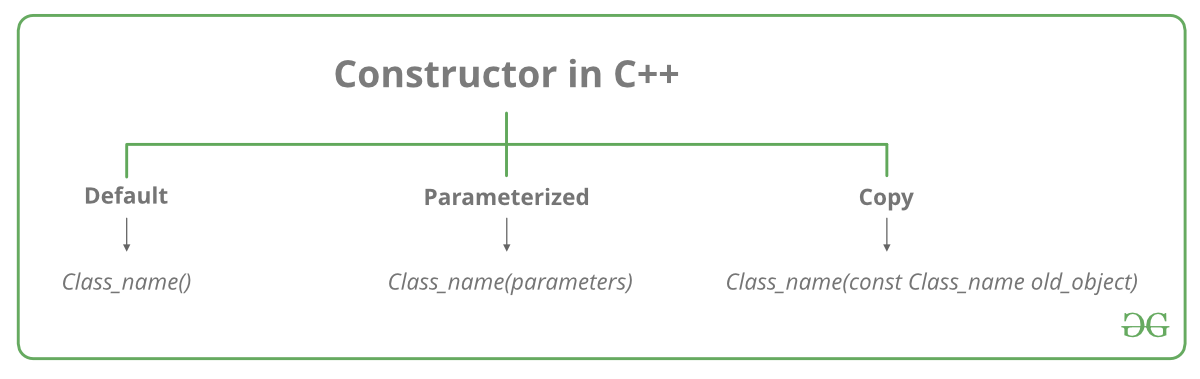# Constructors in C++

What is constructor?
A constructor is a member function of a class which initializes objects of a class. In C++, Constructor is automatically called when object(instance of class) create. It is special member function of the class.
How constructors are different from a normal member function?

A constructor is different from normal functions in following ways:

• Constructor has same name as the class itself
• Constructors don’t have return type
• A constructor is automatically called when an object is created.
• If we do not specify a constructor, C++ compiler generates a default constructor for us (expects no parameters and has an empty body).Let us understand the types of constructors in C++ by taking a real-world example. Suppose you went to a shop to buy a marker. When you want to buy a marker, what are the options? The first one you go to a shop and say give me a marker. So just saying give me a marker mean that you did not set which brand name and which color, you didn’t mention anything just say you want a marker. So when we said just I want a marker so whatever the frequently sold marker is there in the market or in his shop he will simply hand over that. And this is what a default constructor is! The second method you go to a shop and say I want a marker a red in color and XYZ brand. So you are mentioning this and he will give you that marker. So in this case you have given the parameters. And this is what a parameterized constructor is! Then the third one you go to a shop and say I want a marker like this(a physical marker on your hand). So the shopkeeper will see that marker. Okay, and he will give a new marker for you. So copy of that marker. And that’s what copy constructor is!
Types of Constructors

1. Default Constructors: Default constructor is the constructor which doesn’t take any argument. It has no parameters.

## CPP

 `// Cpp program to illustrate the` `// concept of Constructors` `#include ` `using` `namespace` `std;`   `class` `construct ` `{` `public``:` `    ``int` `a, b;`   `    ``// Default Constructor` `    ``construct()` `    ``{` `        ``a = 10;` `        ``b = 20;` `    ``}` `};`   `int` `main()` `{` `    ``// Default constructor called automatically` `    ``// when the object is created` `    ``construct c;` `    ``cout << ``"a: "` `<< c.a << endl` `         ``<< ``"b: "` `<< c.b;` `    ``return` `1;` `}`

Output:

```a: 10
b: 20
```

Note: Even if we do not define any constructor explicitly, the compiler will automatically provide a default constructor implicitly.

2. Parameterized Constructors: It is possible to pass arguments to constructors. Typically, these arguments help initialize an object when it is created. To create a parameterized constructor, simply add parameters to it the way you would to any other function. When you define the constructor’s body, use the parameters to initialize the object.

## CPP

 `// CPP program to illustrate` `// parameterized constructors` `#include ` `using` `namespace` `std;`   `class` `Point ` `{` `private``:` `    ``int` `x, y;`   `public``:` `    ``// Parameterized Constructor` `    ``Point(``int` `x1, ``int` `y1)` `    ``{` `        ``x = x1;` `        ``y = y1;` `    ``}`   `    ``int` `getX()` `    ``{` `        ``return` `x;` `    ``}` `    ``int` `getY()` `    ``{` `        ``return` `y;` `    ``}` `};`   `int` `main()` `{` `    ``// Constructor called` `    ``Point p1(10, 15);`   `    ``// Access values assigned by constructor` `    ``cout << ``"p1.x = "` `<< p1.getX() << ``", p1.y = "` `<< p1.getY();`   `    ``return` `0;` `}`

Output:

```p1.x = 10, p1.y = 15
```

When an object is declared in a parameterized constructor, the initial values have to be passed as arguments to the constructor function. The normal way of object declaration may not work. The constructors can be called explicitly or implicitly.

``` Example e = Example(0, 50); // Explicit call

Example e(0, 50);           // Implicit call
```
1. Uses of Parameterized constructor:
1. It is used to initialize the various data elements of different objects with different values when they are created.
2. It is used to overload constructors.

Can we have more than one constructor in a class?

1. Copy Constructor: A copy constructor is a member function which initializes an object using another object of the same class. Detailed article on Copy Constructor.

Whenever we define one or more non-default constructors( with parameters ) for a class, a default constructor( without parameters ) should also be explicitly defined as the compiler will not provide a default constructor in this case. However, it is not necessary but it’s considered to be the best practice to always define a default constructor.

## CPP

 `// Illustration` `#include "iostream"` `using` `namespace` `std;`   `class` `point ` `{` `private``:` `  ``double` `x, y;`   `public``:` `  `  `  ``// Non-default Constructor & ` `  ``// default Constructor` `  ``point (``double` `px, ``double` `py) ` `  ``{` `    ``x = px, y = py;` `  ``}` `};`   `int` `main(``void``) ` `{`   `  ``// Define an array of size ` `  ``// 10 & of type point` `  ``// This line will cause error` `  ``point a;`   `  ``// Remove above line and program ` `  ``// will compile without error` `  ``point b = point(5, 6);` `}`

Output:

```Error: point (double px, double py): expects 2 arguments, 0 provided
```

Related Articles :

Rated as one of the most sought after skills in the industry, own the basics of coding with our C++ STL Course and master the very concepts by intense problem-solving.

My Personal Notes arrow_drop_up

Article Tags :
Practice Tags :

59

Please write to us at contribute@geeksforgeeks.org to report any issue with the above content.